# NCERT Solutions For Class 9 Maths Chapter 1 Exercise 1.4 – Number System

Download NCERT Solutions For Class 9 Maths Chapter 1 Exercise 1.4 – Number System. This Exercise contains 2 questions, for which detailed answers have been provided in this note. In case you are looking at studying the remaining Exercise for Class 9 for Maths NCERT solutions for Chapter 1 or other Chapters, you can click the link at the end of this Note.

### NCERT Solutions For Class 9 Maths Chapter 1 Exercise 1.4 – Number System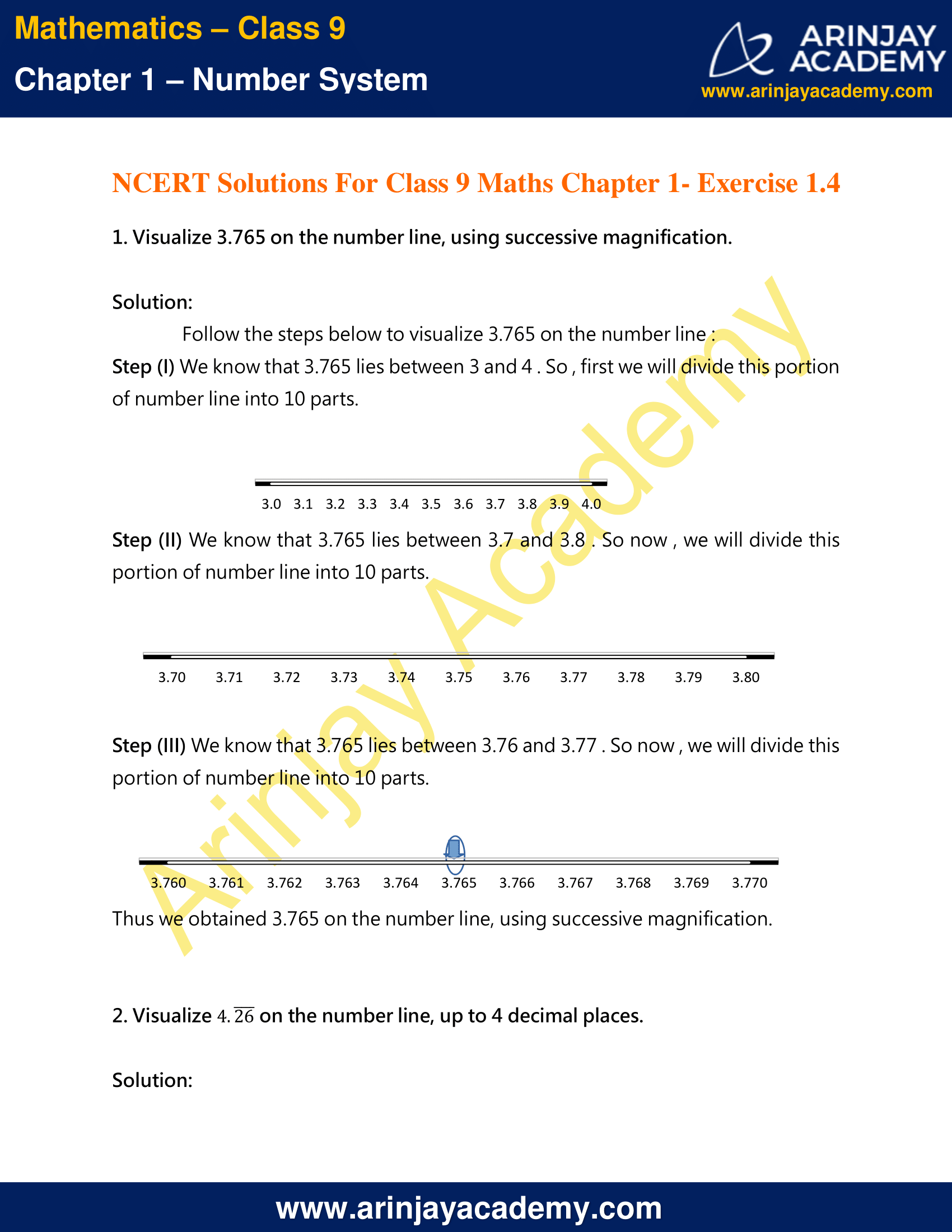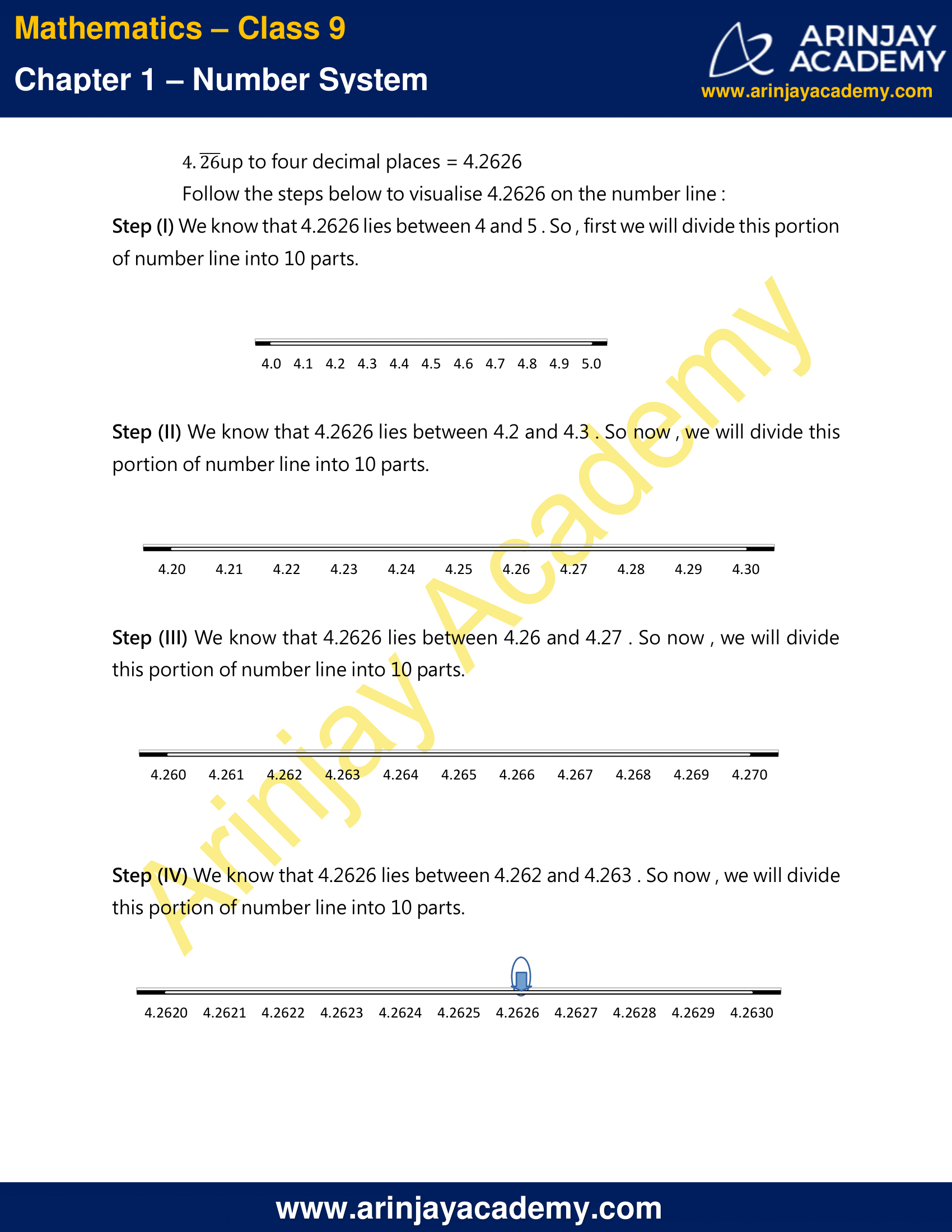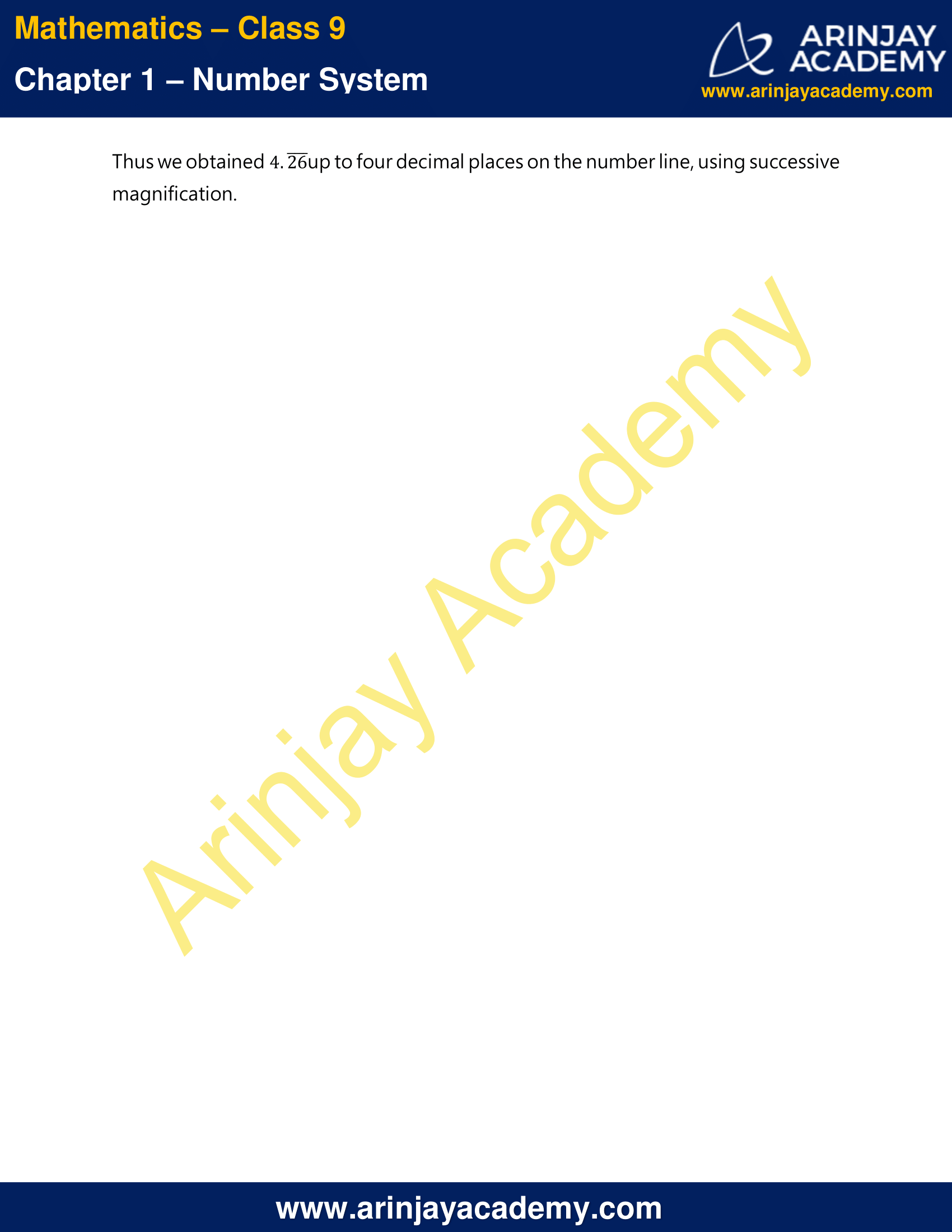NCERT Solutions For Class 9 Maths Chapter 1 Exercise 1.4 – Number System

1. Visualise 3.765 on the number line, using successive magnification.

Solution:

Follow the steps below to visualise 3.765 on the number line :
Step (I) We know that 3.765 lies between 3 and 4 . So , first we will divide this portion of number line into 10 parts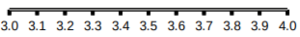Step (II) We know that 3.765 lies between 3.7 and 3.8 . So now , we will divide this portion of number line into 10 parts.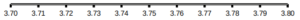Step (III) We know that 3.765 lies between 3.76 and 3.77 . So now , we will divide this portion of number line into 10 parts.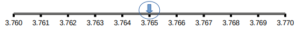Thus we obtained 3.765 on the number line, using successive magnification.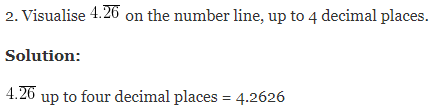Follow the steps below to visualise 4.2626 on the number line :
Step (I) We know that 4.2626 lies between 4 and 5 . So , first we will divide this portion of number line into 10 parts.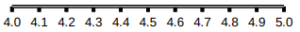Step (II) We know that 4.2626 lies between 4.2 and 4.3 . So now , we will divide this portion of number line into 10 parts.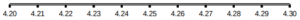Step (III) We know that 4.2626 lies between 4.26 and 4.27 . So now , we will divide this portion of number line into 10 parts.Step (IV) We know that 4.2626 lies between 4.262 and 4.263 . So now , we will divide this portion of number line into 10 parts.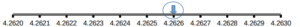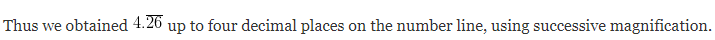NCERT Solutions for Class 9 Maths Chapter 1 Exercise 1.4 – Number System, has been designed by the NCERT to test the knowledge of the student on the topic – Representing Real Numbers on the Number Line

The next Exercise for NCERT Solutions for Class 9 Maths Chapter 1 Exercise 1.5 – Number System can be accessed by clicking here.

Download NCERT Solutions For Class 9 Maths Chapter 1 Exercise 1.4 – Number System